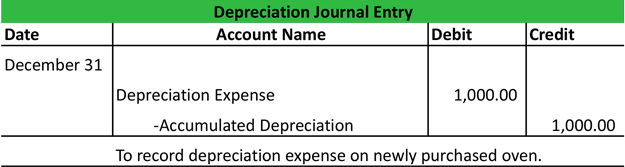# Depreciation Journal Entry

A depreciation journal entry is used at the end of each period to record the fixed asset or plant asset depreciation in the accounting system.

Unlike journal entries for normal business transactions, the deprecation journal entry does not actually record a business event. Instead, it records the passage of time and the use of an asset.

According to the matching principle, long-term assets or capital assets can’t be expensed immediately when they are purchased because their useful life is longer than one year. This makes sense because the company will have a benefit from these assets in future years, so they should also realize expenses in futures that match the benefits. That is why capital assets must be capitalized and depreciated on a systematic and consistent basis.

It’s a common misconception that depreciation is a form of expensing a capital asset over many years. Depreciation is really the process of devaluing the capital asset over a period of time due to age and use. Depreciation and accumulated depreciation shows the current value or book value of the used asset.

The depreciation journal entry records depreciation expense as well as accumulated depreciation. Depreciation expense is debited for the current depreciation amount and accumulated depreciation is credited. The depreciation expense is then presented on the income statement as an operating expense and the accumulated depreciation is presented on the balance sheet as a contra capital asset account.

There are many different depreciation methods and rates, but we will use the straight-line deprecation method for this example. The straight-line depreciation method computes depreciation expense like this: depreciation expense = (asset purchase price – salvage value) / useful life.

## Depreciation Journal Entry Example

Big John’s Pizza, LLC bought a new pizza oven at the beginning of this year for \$10,000. Big John, the owner, estimates that this oven will last about 10 years and probably won’t be worth anything after 10 years. At the end of the year, Big John would record this depreciation journal entry.Depreciation for the year was calculated on the straight-line method. Since the oven had no salvage value, the depreciation expense for the year is simply \$10,000 divided by 10 years or \$1,000 per year.